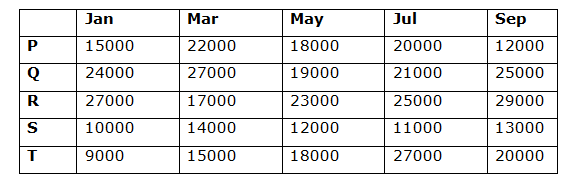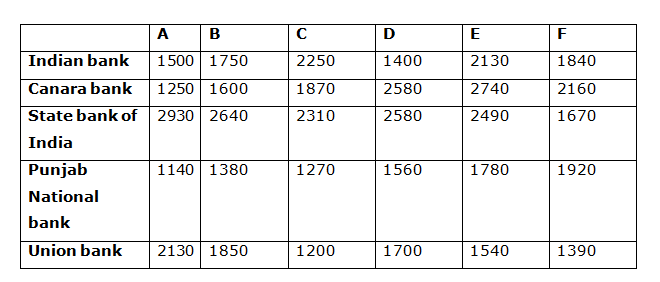# Quantitative Aptitude Questions (Data Interpretation) for SBI Clerk/PO Prelims 2018 Day-144

Dear Readers, SBI is conducting Online Examination for the recruitment of Clerical Cadre and probationary officer. To enrich your preparation here we have providing new series of Data Interpretation – Quantitative Aptitude Questions. Candidates those who are appearing in SBI Clerk/PO Prelims Exams can practice these Quantitative Aptitude average questions daily and make your preparation effective.

[WpProQuiz 2552]

Click “Start Quiz” to attend these Questions and view Solutions

Directions (Q. 1 – 5) Study the following information carefully and answer the given questions:

Following table shows the electricity amount (In Rs.) of different months in the year 2017 of 5 different offices.1. The electricity amount of the office P, R and T together in the month of Mar and Jul is approximately what percentage of the electricity amount of the office Q, S and T together in the month of Jan and Sep?
1. 112 %
2. 125 %
3. 98 %
4. 76 %
5. 134 %
1. Find the difference between the total electricity amount of office P of all the given months together to that of the total electricity amount of office T of all the given months together?
1. 2000
2. 3500
3. 4000
4. 1500
5. None of these
1. Find the ratio between the total electricity amount of Q and T in the month of Jan, Mar and May together to that of total electricity amount of R and S in the month of May, July and Sep together?
1. 56 : 59
2. 43 : 45
3. 37 : 33
4. 112 : 113
5. None of these
1. Find the average electricity amount collected in the month of May and July together from all the given offices?
1. 97900
2. 92500
3. 97000
4. 92700
5. None of these
1. The electricity amount of the office Q and S together in the month of Jan and Sep is approximately what percentage more/less than the electricity amount of the office R and T together in the month of Mar and July?
1. 14 % less
2. 27 % more
3. 14 % more
4. 27 % less
5. 35 % more

Directions (Q. 6 – 10) Study the following information carefully and answer the given questions:

Following table shows the total number of application received for six different specialist posts by five different banks in a year.1. Total number of application received for a specialist post A, C and E together in Indian bank and State bank of India is approximately what percentage of total number of application received for a specialist post B, D and F together in Canara bank and Punjab National bank ?
1. 168 %
2. 152 %
3. 146 %
4. 122 %
5. 115 %
1. Find the ratio between the total number of application received for all the given specialist post together in Canara bank to that of Union bank?
1. 356 : 115
2. 1220 : 981
3. 1331 : 452
4. 457 :123
5. None of these
1. Find the difference between the total number of application received for Specialist post C to that of Specialist post E from all the given banks together?
1. 1780
2. 2540
3. 3130
4. 2950
5. None of these
1. Which of the following bank has received least number of applications for all the given specialist post together?
1. Indian bank
2. Canara bank
3. Punjab National bank
4. Union bank
5. State bank of India
1. Find the sum of application received for the specialist post C and F all the banks together
1. 23560
2. 17880
3. 29720
4. 14370
5. None of these

The electricity amount of the office P, R and T together in the month of Mar and Jul

= > 22000 + 20000 + 17000 + 25000 + 15000 + 27000

= > 126000

The electricity amount of the office Q, S and T together in the month of Jan and Sep

= > 24000 + 25000 + 10000 + 13000 + 9000 + 20000

= > 101000

Required % = (126000/101000)*100 = 124.75 % = 125 %

The total electricity amount of office P of all the given months together

= > 15000 + 22000 + 18000 + 20000 + 12000

= > 87000

The total electricity amount of office T of all the given months together

= > 9000 + 15000 + 18000 + 27000 + 20000

= > 89000

Required difference = 89000 – 87000= 2000

The total electricity amount of Q and T in the month of Jan, Mar and May together

= > 24000 + 27000 + 19000 + 9000 + 15000 + 18000

= > 112000

The total electricity amount of R and S in the month of May, July and Sep together

= > 23000 + 25000 + 29000 + 12000 + 11000 + 13000

= > 113000

Required ratio = 112000: 113000 = 112: 113

The total electricity amount collected in the month of May and July together from all the given offices

= > 18000 + 19000 + 23000 + 12000 + 18000 + 20000 + 21000 + 25000 + 11000 + 27000

= > 194000

Required average = 194000/5 = 38800

The electricity amount of the office Q and S together in the month of Jan and Sep

= > 24000 + 25000 + 10000 + 13000

= > 72000

The electricity amount of the office R and T together in the month of Mar and July

= > 17000 + 25000 + 15000 + 27000

= > 84000

Required % = [(84000 – 72000)/84000]*100 = 14.28 % = 14 % less

Directions (Q. 6 – 10)

Total number of application received for a specialist post A, C and E together in Indian bank and State bank of India

= > 1500 + 2250 + 2130 + 2930 + 2310 + 2490 = 13610

Total number of application received for a specialist post B, D and F together in Canara bank and Punjab National bank

= > 1600 + 2580 + 2160 + 1380 + 1560 + 1920 = 11200

Required % = (13610/11200)*100 = 121.5 % = 122 %

The total number of application received for all the given specialist post together in Canara bank

= > 1250 + 1600 + 1870 + 2580 + 2740 + 2160 = 12200

The total number of application received for all the given specialist post together in Union bank

= > 2130 + 1850 + 1200 + 1700 + 1540 + 1390 = 9810

Required ratio = 12200: 9810 = 1220: 981

The total number of application received for Specialist post C from all the given banks together

= > 2250 + 1870 + 2310 + 1270 + 1200 = 8900

The total number of application received for Specialist post E from all the given banks together

= > 2130 + 2740 + 2490 + 1780 + 1540 = 10680

Required difference = 10680 – 8900 = 1780

Indian bank

= > 1500 + 1750 + 2250 + 1400 + 2130 + 1840 = 10870

Canara bank

= > 1250 + 1600 + 1870 + 2580 + 2740 + 2160 = 12200

State bank of India

= > 2930 + 2640 + 2310 + 2580 + 2490 + 1670 = 9640

Punjab National bank

= > 1140 + 1380 + 1270 + 1560 + 1780 + 1920 = 9050

Union bank

= > 2130 + 1850 + 1200 + 1700 + 1540 + 1390 = 9810

Punjab National bank has received least number of applications.

The sum of application received for the specialist post C and F together from all the banks

= > 2250 + 1870 + 2310 + 1270 + 1200 + 1840 + 2160 + 1670 + 1920 + 1390

= > 17880

Daily Practice Test Schedule | Good Luck

 Topic Daily Publishing Time Daily News Papers & Editorials 8.00 AM Current Affairs Quiz 9.00 AM Quantitative Aptitude “20-20” 11.00 AM Vocabulary (Based on The Hindu) 12.00 PM General Awareness “20-20” 1.00 PM English Language “20-20” 2.00 PM Reasoning Puzzles & Seating 4.00 PM Daily Current Affairs Updates 5.00 PM Data Interpretation / Application Sums (Topic Wise) 6.00 PM Reasoning Ability “20-20” 7.00 PM English Language (New Pattern Questions) 8.00 PM